# Difference between revisions of "Coriolis and tidal motion in shelf seas"

## Introduction

Tides are generated in the ocean in response to the gravitational attraction of moon and sun. Ocean tides generate tidal motion in the shelf seas. Tidal motion in the shelf seas differs from the ocean tide due to the much smaller water depth and the presence of land boundaries. In this article some characteristics of tidal motion in shelf seas are discussed, complementing the more general introduction in the article Ocean and shelf tides. Although the discussion is restricted to simple geometries – uniform depth and straight coastlines – the general characteristics are similar to tidal motion in shelf seas with more complex geometries.

Tidal waves in wide basins are strongly influenced by earth's rotation (Coriolis acceleration). Tidal waves turn around so-called amphidromic points, where the vertical motion is almost nil. At some distance from the basin boundaries the tidal current vector describes an ellipse during the tidal period.

## Assumptions

We will restrict the discussion to situations where the following assumptions approximately hold:

• a shelf sea of uniform depth and uniform density;
• a single (semi-diurnal) tidal component with radial frequency $\omega$ ;
• tidal motion driven by the ocean tide at the shelf boundary;
• tide-generating forces of moon and sun are negligible for the selected domain;
• latitudinal variation of the Coriolis parameter $f$ can be neglected;
• frictionless tidal flow;
• tidal amplitude much smaller than water depth;
• current velocities much smaller than tidal wave celerity $c$.

## Tidal equations

With the above assumptions, the tidal equations (momentum balance and mass balance) including coriolis acceleration $f=2\Omega \sin \phi$, can be written as:

$\Large \frac{\partial u}{\partial t} \normalsize - f v + g \Large \frac{\partial \eta}{\partial x} \normalsize =0 , \qquad (1)$

$\Large \frac{\partial v}{\partial t} \normalsize + f u + g \Large \frac{\partial \eta}{\partial y} \normalsize =0 , \qquad (2)$

$\Large \frac{\partial \eta}{\partial t} \normalsize + h \; ( \Large \frac{\partial u}{\partial x} + \Large \frac{\partial v}{\partial y} \normalsize ) =0 . \qquad (3)$

The following conventions are used:

• $u,v$ are the depth-averaged tidal current velocities in $x,y$-directions, respectively;
• $\eta$ is the tide level;
• $h$ is the average depth;
• $\Omega$ is the radial frequency of earth's rotation;
• $\phi$ is the latitude expressed in radians (positive for the northern hemisphere, negative for the southern hemisphere).

The current velocities $u,v$ can be eliminated from the tidal equations (1-3); this gives the wave equation

$\Large \frac{\partial^2 \eta }{\partial t^2} \normalsize + f^2 \eta = gh \; (\Large \frac{\partial^2 \eta }{\partial x^2} + \frac{\partial^2 \eta }{\partial y^2} \normalsize ) . \qquad (4)$

## Dispersion relationFigure 1: At some distance from the coastal boundaries the tidal current vector describes an ellipse during the tidal cycle. The current vector runs the ellipse in clockwise direction on the northern hemisphere.

The solution of equation (4) for a propagating tidal wave has the form

$\eta(x,y,t) = A \cos(kx+my-\omega t) e^{\large -\kappa x - \mu y \normalsize}. \qquad (5)$

The propagation direction is given by the vector $\vec k = (k,m)$, and the phase speed is given by $c=\omega / \sqrt{k^2+m^2} .$ Substitution of this solution yields the dispersion relation

$gh(k^2+m^2-\kappa^2-\mu^2+2i(k \kappa +m \mu))=\omega^2-f^2 . \qquad (6)$

By choosing the $x$-axis along the propagation direction (i.e. $m=0, \; k=\omega / c$), the dispersion relation requires $\kappa =0$ and

$gh(k^2-\mu^2)=\omega^2-f^2 . \qquad (7)$

For the tidal wave solution the corresponding velocity components are given by

$u = g \Large \frac{k \omega - \mu f }{\omega^2-f^2} \normalsize \eta , \; v = g \Large \frac{k f - \mu \omega}{\omega^2-f^2} \normalsize \eta \tan(kx-\omega t). \qquad (8)$

At $x=0$ the velocity components are

$u = U (1 - \Large \frac{\mu f c}{\omega^2} \normalsize) \cos \omega t, \; v = - U \Large \frac{f -\mu c}{\omega} \normalsize \sin \omega t , \; U = c \Large \frac{A/h} {1 -(\mu c / \omega)^2} \normalsize e^{\large -\mu y \normalsize }. \qquad (9)$

For $\mu c \lt f \lt \omega ,$ the current vector ($u,v$) rotates anti-cyclonically around an ellipse; for $\mu=0$ the demi-axes are ($A \omega / kh, A|f|/kh$), see figure 1.

## Kelvin wave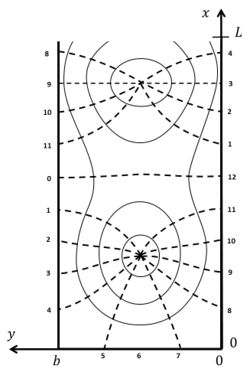Figure 2: Co-tidal lines (indicated by dashed lines, with phase hour) and lines of equal amplitude (solid lines) according to the solution of G.I. Taylor, for tidal propagation in a rectangular semi-enclosed basin with uniform depth. The dots indicate the amphidromic points.

A particular solution for a tidal wave propagating in positive $x$-direction along the coast corresponds to

$\mu = f/c , \; c=\sqrt{gh} . \qquad (10)$

For this solution, which is called Kelvin wave, we have $v=0$ (tidal velocity parallel to the shoreline) and

$\eta(x,y,t)= A e^{\large-fy/c \normalsize } \cos(kx-\omega t) ,\; u= (Ac/h) e^{\large -fy/c \normalsize } \cos(kx-\omega t). \qquad (11)$

The Kelvin wave is a reasonable approximation for tidal waves in the vicinity of a straight coastline $y=y_0$, where $v \approx 0$.

The Kelvin wave is largest near the coastline and exponentially damped further away; it propagates along the coast with the coastline at the right hand in the northern hemisphere ($f,y\gt y_0$) and with the coastline at the left hand in the southern hemisphere ($f,y\lt y_0$). For a Kelvin wave propagating in negative $x$-direction along the coast (northern hemisphere) $\mu = -f/c$.

## Amphidromic points

A tidal wave propagating in a rectangular basin of length $L$ can be described by the sum of two Kelvin waves. In the northern hemisphere (figure 2) we have an incoming wave propagating in negative $x$-direction along the left boundary $y=b$ and a reflected wave propagating in positive $x$-direction along the right boundary $y=0$,

$\eta = (a/2) \left[e^{\large f(y-b/2)/c \normalsize }\cos(kx+\omega t) + e^{\large -f(y-b/2)/c \normalsize } \cos(kx-\omega t) \right] , \qquad (12)$

$u = (ca/2h) \left[- e^{\large f(y-b/2)/c \normalsize }\cos(kx+\omega t) + e^{\large -f(y-b/2)/c \normalsize } \cos(kx-\omega t) \right] . \qquad (13)$

The closed landward boundary is at $x=0$ and the open seaward boundary at $x=L$. At $x=\pi/2k, y=b/2$ the vertical wave motion is zero; this is the so-called amphidromic point. In long basins another amphidromic point exists at $x=3\pi/2k, y=b/2$. In long wide basins the tidal wave composed of incoming and reflected Kelvin waves turns around amphidromic points, see figure 2.

In the ocean the semidiurnal lunar tidal wave turns around amphidromic points, see figure 3. This illustrates that ocean tides has the characteristics of a system of Kelvin waves.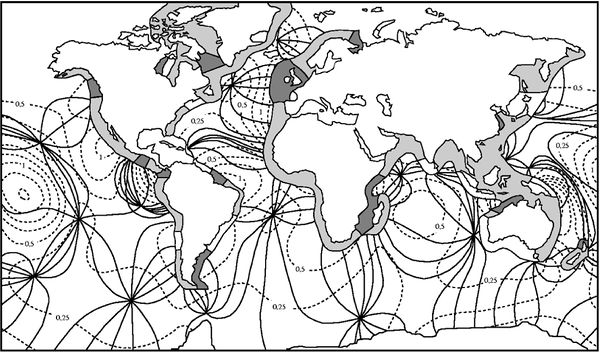Figure 3: Co-tidal lines (solid lines) and lines of equal amplitude (dashed lines, amplitude in meters) of the semidiurnal lunar M2-tide in the oceans. The tidal range along the coast is indicated in dark for tidal ranges larger than 4 m, in grey for tidal ranges between 2 and 4 m and in white for tidal ranges below 2 m.

## Boundary conditions

We consider a tidal basin with a closed end at $x=0$. The width $b$ is much smaller than the tidal wavelength $2 \pi \sqrt{gh}/\omega$, which is smaller than the basin length $L$. Because Kelvin waves are not a full solution of the tidal equations, the condition $u=0$ at the closed end is satisfied only for $y=b/2$. Other wave solutions have to be taken into account near the closed end of the basin. These are standing waves, sometimes called Poincaré waves. They are periodic in the lateral coordinate $y$ in order to satisfy the condition $v=0$ at the lateral basin boundaries $y=(0,b)$,

$v = \sum_{n=1}^{n=\infty} v_n, \quad v_n = V_n e^{\large - p_n x \normalsize } \sin (\large \frac{n \pi y }{b} \normalsize) \cos(\omega t) . \qquad (14)$

According to this equation, the spatial and temporal dependence of the tidal elevation and tidal velocities is represented by a series of functions

$\eta, \; u, \; v \sim \exp(p_n x) \exp(i \large\frac{n \pi y}{b}\normalsize) \exp(i \omega t) . \qquad (15)$

Substitution in equation (4) gives the dispersion relation

$p_n^2 =(\Large \frac{n\pi}{b})^2- \frac{\omega^2-f^2}{gh} \normalsize . \qquad (16)$

In basins which are much narrower than the tidal wavelength, $n \pi /b \gt \gt \omega / \sqrt{gh}$, the exponents $p_n$ are real positive numbers, $p_n \approx n \pi /b$. The Poincaré waves are significant only near the end of the basin and strongly damped elsewhere. The contribution of the Poincaré waves to the velocity $u$ can be derived by substitution of the expressions (15) in the equations (1, 2).

The coefficients $V_n$ are determined from the condition

$u_K(0,y,t)+u_P(0,y,t)=0$

at the closed boundary $x=0$. The subscripts $K,P$ indicate the Kelvin and Poincaré wave contributions, respectively. A first order approximate solution is

$u_K= V_1 e^{\large - p_1 x \normalsize } \cos (\large \frac{ \pi y }{b} \normalsize) \cos(\omega t) , \qquad V_1=-(A/h)bf/\pi .$

The Poincaré wave solution for satisfying the seaward boundary condition (the ocean tide at $x=L$) can be found in a similar way. An analytical solution for the problem of tidal motion in a rectangular basin was first given by G.I. Taylor in 1921 , as shown in figure 2.

## Influence of friction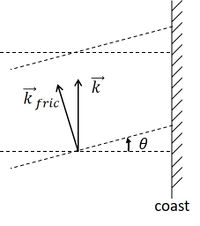Figure 4: Rotation of the propagation direction of a Kelvin wave away from the coast due to bottom friction: $\vec k \; \to \; \vec k_{fric}$. Bottom friction rotates the crestlines (dotted lines) of a Kelvin wave in cyclonic direction.

Inclusion of friction influences the tidal wave in several important ways. Analytical expressions can be derived if the tidal equations are linearised. Because calculations are quite lengthy, the reader is referred to the literature , . Here we only mention some major consequences of the inclusion of friction terms in the tidal equations.

(1) The crests of Kelvin waves are not perpendicular to the coastline, but are rotated in cyclonic direction with respect to the propagation direction, see figure 4. The wave propagation direction is away from the coast.

(2) The amphidromic points are not situated on the center line of semi-enclosed basins, but they are shifted in cyclonic direction relative to the propagation direction of the incoming tidal wave, see figure 5.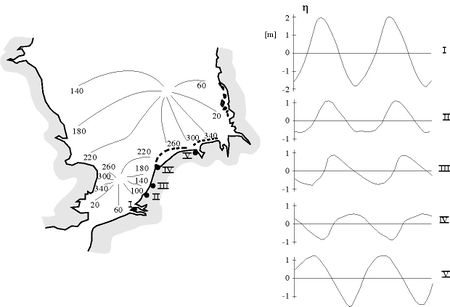Figure 5:Left panel: Co-tidal lines of the M2-tidal wave in the North Sea. The tidal wave enters mainly from the north, follows the UK coast at the right hand and returns along the Dutch, German and Danish coast. The amphidromic point in the central North Sea is shifted cyclonically with respect to the propagation direction of the incoming tidal wave. In the southern North Sea, the tide is influenced by the tidal wave entering through the Channel; this explains why the amphidromic point is not shifted toward the Dutch coast. Right panel: Tide curves along the Dutch coast at locations I-V. Tidal asymmetry (faster tidal rise and slower tidal fall) increases along the propagation direction (from I-IV). At location V the asymmetry has vanished, because the tide is mainly determined by the amphidromic system of the central North Sea.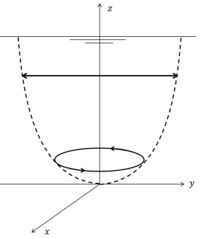Figure 6: Transformation of a rectilinear tidal current near the surface to an elliptic tidal current near the bottom, by the combined influence of Coriolis acceleration and bottom friction.

(3) The current velocity vector rotates in cyclonic direction with increasing distance from the water surface ,, see figure 6. This can be shown by decomposing the tidal current into two circular rotating components, one component rotating in cyclonic direction and the other component in anticyclonic direction. If the magnitude of the two components is the same, the tidal current is rectilinear. Frictional damping depends on the rotation sense. The anticyclonic component is more strongly damped with increasing depth than the cyclonic component; the latter therefore dominates near the bottom.

(4) In shallow coastal zones, with significant tide compared to the depth, the Kelvin wave propagates faster at high tide than at low tide. The tidal wave therefore becomes increasingly distorted in the propagation direction; tidal rise becomes faster than fall (see for an explanation: Tidal asymmetry and tidal basin morphodynamics). This is illustrated in figure 5 for the tidal wave propagating along the Dutch coast. Fast tidal rise causes stronger flood currents than ebb currents in adjacent tidal lagoons and estuaries. This tidal asymmetry stimulates sediment import. It can result in silting up of lagoons and in the formation of turbidity maxima in estuaries.

## Related articles

Ocean and shelf tides
Coriolis acceleration
Tidal asymmetry and tidal basin morphodynamics